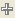Publication date: 04/12/2021

## Evaluate Formulas

By default, JMP evaluates each formula that you create. You can turn this evaluation off, or you can use it before you have finished creating a formula.

### Suppress Evaluation

Turning off evaluation is a useful formula development mode for building complex formulas. You can turn off evaluation and build sections of a formula, and evaluate only to test it. In particular, you can close the Formula Editor and reopen it at a later time to continue building a formula without JMP evaluating it.

To suppress formula evaluation, click the red triangle next to the keypad and select Suppress Eval. When evaluation is suppressed, the formula iconappears dimmed.

Note the following:

If the icon appears to the right of the red triangle in the Formula Editor, it indicates that formula evaluation is suppressed for that formula.

If the icon appears beside the column name in the Columns panel, it indicates that the values in the column result from a formula. When formula evaluation is suppressed, this formula iconappears dimmed. See Icons Representing Column Characteristics and Properties.

To turn off formula evaluation for all formulas, click the red triangle next to the data table name and select Suppress Formula Eval. To re-evaluate formulas, click the red triangle next to the data table name and select Rerun Formulas. You can also click the Apply button in the Formula Editor for each column that contains a pending formula.

When you turn off formula evaluation, edit a formula, and click Apply, you are prompted for whether to evaluate the formula. To allow formula evaluation once, click Evaluate formula this time.

Want more information? Have questions? Get answers in the JMP User Community (community.jmp.com).